Thank you for visiting nature.com. You are using a browser version with limited support for CSS. To obtain the best experience, we recommend you use a more up to date browser (or turn off compatibility mode in Internet Explorer). In the meantime, to ensure continued support, we are displaying the site without styles and JavaScript.

# Predicting commuter flows in spatial networks using a radiation model based on temporal ranges

## Abstract

Understanding network flows such as commuter traffic in large transportation networks is an ongoing challenge due to the complex nature of the transportation infrastructure and human mobility. Here we show a first-principles based method for traffic prediction using a cost-based generalization of the radiation model for human mobility, coupled with a cost-minimizing algorithm for efficient distribution of the mobility fluxes through the network. Using US census and highway traffic data, we show that traffic can efficiently and accurately be computed from a range-limited, network betweenness type calculation. The model based on travel time costs captures the log-normal distribution of the traffic and attains a high Pearson correlation coefficient (0.75) when compared with real traffic. Because of its principled nature, this method can inform many applications related to human mobility driven flows in spatial networks, ranging from transportation, through urban planning to mitigation of the effects of catastrophic events.

## Introduction

One of the challenges in network science is predicting network flows from graph structural properties, node/edge attributes and dynamical rules. While for some networks (for example, electronic circuits) this is a well-understood problem, it is still open in general, and especially for networks involving a social component1,2 such as communication networks3,4, epidemic networks5,6,7 and infrastructure networks8,9,10,11,12,13,14,15,16,17,18,19. Here we focus on the traffic flow prediction problem in spatial networks, and in particular in roadway networks, and validate our results using US highway network and traffic data (http://libguides.mit.edu/gis). Understanding flows in spatial networks driven by human mobility would have many important consequences: it would enable us to connect throughput properties with demographic factors and network structure; it would inform urban planning20,21,22,23; help forecast the spatio-temporal evolution of epidemic patterns5,6,7, help assess network vulnerabilities24,25 and allow the prediction of changes in the wake of catastrophic events26.

When modelling transportation systems as networks, we usually associate network nodes with locations and edges with physical paths between locations. Here, we define nodes as intersections between the roads and the road segment between two consecutive intersections as the edge connecting those nodes. We will refer to nodes also as sites or locations, interchangeably. Our ultimate goal is to determine the average traffic flow Tij expressing the number of flow units (for example vehicles) per unit time (for example per day) through an edge (i, j) of the network, given the network and the distribution of the population.

For any traffic to exist, there must be people planning to travel between locations. Given an origin location a and destination b, the average number of travellers from a to b is determined by socio-demographic factors such as distribution of the population, availability of jobs, resource locations and so on. We define Φab as the average number of daily travellers planning to go from site a (origin) to site b (destination), where the average is computed over a longer time interval such as a year period. We call Φab the mobility flux, or origin–destination (OD) flux, and use the word flux exclusively for that purpose. The socio-demographic model that describes the fluxes Φab will be called mobility law. Note that the flux Φab does not tell us anything about the path chosen between the origin and destination. It is simply the size of population at location a planning to travel daily to location b. When people travel from a location a to a location b they must choose a route on the network to do so. Accordingly, the Tij expresses the average number of daily travellers through edge (i, j), which can in principle originate from any location a travelling to any location b as long as their chosen route on the network contains the road segment (i, j). When referring to traffic on specific edges (road segments), that is the Tij-s, we will use the word flow, or traffic interchangeably. Note that Φab is well defined for any two nodes or locations a and b in the network, but it does not define any traffic (flow); whereas Tij is defined only for edges (i, j) and it is a flow quantity. In analogy with physics Φab corresponds to voltage, whereas Ti,j corresponds to current.

Modelling traffic flows in spatial networks can therefore be approached via solving two problems: (1) determining the mobility fluxes Φab for all OD pairs (a,b)27,28,29 and (2) distributing the fluxes Φab through the network, that is determining the network paths along which the flow units are transported11,12,14,15. We call the first problem the mobility law problem and the second the flux distribution problem and present a solution to both problems in this paper.

The common approach to the mobility law problem has been through the use of gravity models3,11,16,28,29,30,31, which assume that the fluxes have the generic form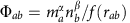where ma and nb are the population sizes of origin a and destination b, rab is the distance between them, and f(x) is called the deterrence function. Typical forms for f are power-law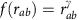or exponential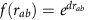, where α, β γ and d are fitting parameters. As shown in ref. 27 gravity models are essentially fitting forms and they have numerous ills. Besides not being based on first principles, the fitting parameters can vary wildly even within a single data set (as function of rab)3,7,30,31,32. They can also show non-physical behaviour, for example, when the destination has a large enough population, the number of travellers can exceed the size of the origin population. Recently, a novel mobility law called the radiation model was introduced using probabilistic arguments, which avoids the problems of gravity models27,33. Here we will use the radiation model as the mobility law with a first-principles-based generalization that allows us to couple it with the network structure, where mobility takes place.

Given the Φab fluxes for all the N(N−1) node pairs (a, b) obtained from the generalized mobility law, here we solve the flux distribution problem by using a cost-minimization principle, based on the expectation that commuters tend to minimize the cost of travel. This results in a novel, efficient capacity-aware flux distribution algorithm that helps predict traffic in roadway networks.

## Results

The averaging in the definition of the flux Φab reduces the effect of fluctuations due to seasonal and occasional travel, and thus it is expected to be determined mainly by travellers who commute regularly between home locations and job sites and regular freight traffic. The radiation model is a socio-demographic model27 based on the assumption that people will search for the closest job opportunity that meets their expectation (see Supplementary Note 1). The expectation of an individual is modelled by a single variable z called the benefit variable, which acts as an absorption threshold: an individual ‘emitted’ from location a will take a job at another location b (it becomes absorbed at b) only if the z variable associated with the job site at b surpasses that of the individual’s and she could not find any such absorption site closer than b. Paper27 derives the expression of the probability pab for an individual from location a with population ma to find the closest job opportunity that meets her expectation at location b with population nb and nowhere closer within a range of rab, where rab is the distance between a and b. Assuming independent emission-absorption events, the average mobility flux from a to b is then given by: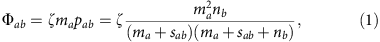where ζ is the fraction of travellers in a location, considered to be an overall constant characterizing the whole of the population and sab is the size of the population within a disc of radius rab centred on a, excluding the populations at locations a and b, see Fig. 1a. The distance rab is interpreted as the crow flies, which, in heterogeneous environments does not usually correspond to the actual length of travel from a to b. Here we extend the radiation model by saying that the individual will be choosing the site b that has the lowest travel cost cab on the network, with a benefit factor at least as large as the individual’s. We will refer to this model as the cost-based radiation model. We compare two travel cost measures, in particular one based on path lengths ab and the other based on travel times τab, both measured along roads. The path length ab is the shortest distance (in km) from a to b along existing network paths, so it is closely related but larger than the geodesical radius rab (measured as great-circle distance). The second travel cost measure is the shortest time (in minutes) τab it takes to go from a to b along the network paths, and thus it depends on travel speeds as well. The expression for the fluxes is still given by (1); however, the population sizes sab are computed differently. Accordingly, the shape of the area around site a with cost of travel not larger than cab on the network is no longer an annular disc with a dent as in Fig. 1a, but it has an amoeboid shape as shown in Fig. 1b. There is an important difference between the criterion rab used in ref. 27 and our general cost criterion cab. The former decouples the mobility law from the underlying transportation network, whereas the cab (hence sab and thus the Φab) depends on the network of paths and their properties, thus coupling the mobility law with the network itself.

### Flux distribution without capacity limitation

The total flow Tij through edge (i, j) is generated by all those travellers that happen to have edge (i, j) on the lowest cost path between their start and end locations. For a pair of OD sites (a, b), let us denote by Pab the set of all network paths from a to b and by ωabεPab a minimal cost path. Thus ωab is a sequence of edges ωab={(a, i2),(i2, i3),…,(iL, b)} such that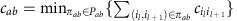is attained for πab=ωab (see Fig. 1c). Note that in principle, there might be several paths with the same lowest cost (called ‘minimal’ paths hereafter) and this possible degeneracy must be included in the expression of the total traffic flow through a given edge (i, j):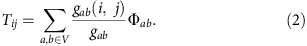Here gab is the number of minimal paths from a to b and gab(i, j) is the number of minimal paths that contain edge (i, j). When the cost cab is not an integer value but a real number (physical distance or travel time), usually there is no degeneracy (gab =1 and gab(i, j) =1 if (i, j) belongs to ωab, zero otherwise) and (2) sums whole fluxes. According to (2), traffic values are obtained from sums of fluxes weighted by adimensional quantities, and thus traffic and flux have the same unit of measure. Realistic traffic data are typically provided in units of vehicles per day in which case we need to multiply the r.h.s. of (2) with an overall constant representing the average number of vehicles per travelling person, here included into ζ, for simplicity. Also for simplicity, we will omit to indicate the unit of measure for fluxes and traffic, showing only numerical values, with the implicit assumption that they are in units of number of vehicles per day.

Equation (2) is similar to the expression of edge betweenness centrality25,34,35,36,37, with the difference being that instead of computing with the number of minimal paths, we now use weights of minimal paths, which are the mobility fluxes computed from the mobility law (the cost-based radiation model in this case). Therefore, the flows Tij can be obtained using the same algorithm as for weighted betweenness centrality25,34,35 with two necessary modifications.

One concerns implementation (see Methods section) and the other exploits the notion of range-limitation. For realistic size networks (infrastructure networks with hundreds of thousands to millions of nodes) the computation of (2) for all edges can become unfeasible (especially for collecting statistics). One can reduce the computational costs by introducing a range-limit on how far (in cost measure) we build the minimal paths tree (MPT) from the source (root) node25,37. In particular we only build the largest MPT from root a such that for all nodes ε in it we have cC. The rationale is that beyond a cost threshold C the contribution of the corresponding mobility fluxes is very small. The full-range algorithm has a complexity of (NM logN), where N is the number of nodes and M is the number of edges. In the case of US highways (sparse network) this is a computation on the order of 1010–1012, which is relatively costly. However, as we show in later sections, for the case of contiguous US, range limitation can reduce this complexity by several orders of magnitude without considerably affecting the accuracy of the results.

### Flux distribution with capacity limitation

Network congestion is a ubiquitous phenomenon, resulting from edges having a finite transmission capacity. We define the transmission capacity Cij of an edge (i, j) as the largest daily flow value above which individuals will choose alternative routes with high probability. Next we show how to distribute the mobility fluxes in a capacity-limited network assuming that all the Cij values are known.

We use dynamic distribution of the traffic by gradually increasing the number of travellers until the first q congested edges appear. The congested edges are then removed from the network for further traffic. More travellers are subsequently added to the network until another q edges become congested, which are then closed for further traffic, and this process is repeated until all travellers have been distributed into the network. Ideally q=1, but it is better to choose q>1 (such as q=100, but still with qM), because on one hand congestion thresholds in finite systems are not sharp and thus q>1 serves as a ‘softness’ parameter, and on the other hand it speeds up the computations.

Let us denote by tij(G) the flow on the edges of a network (or graph) G computed using equation (1) with ζ=1, that is with Φab=mapab. Note that the multiplicative coefficient ζ in the mobility fluxes (1) is also multiplicative in the traffic (or flow) values. Let us denote by Gn the graph obtained from Gn−1 after removing the set Ln of q congested edges in the nth step. We define recursively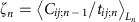with Tij;0≡0, G0=G, where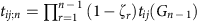is the non-adjusted traffic coming from mobility fluxes Φab corresponding to the fraction of the population not already in the network in that step and Cij;n−1=CijTij;n−1 are the corresponding reduced capacities in Gn. The set Ln is defined as the q edges with the smallest ratios Cij;n−1/tij;n. In the Methods section we show that after k iterations the final flow becomes: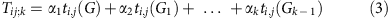where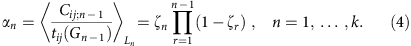The total number of iterations k (stopping criterion) is determined by having all the travelling population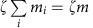distributed onto the network, that is, k is the smallest integer for which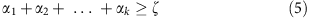holds.

### Comparison with empirical data

To validate our approach we compared the model’s output with real traffic data from a US highway network database (http://libguides.mit.edu/gis), which consists of M=174,753 road segments (edges) and N=137,267 intersections (nodes). The node features are longitude and latitude and the edge features are the IDs of the end nodes, road length, road class, number of lanes and annual average daily traffic (number of vehicles per day). The traffic values are available for about 43% of all edges (road segments) randomly distributed throughout the continental US (see Fig. 2a) providing a good statistical basis for comparisons.

Traffic values were generated for all road segments by the model via equations (2) or (3, 4, 5), following the methods described in the previous sections (also see Supplementary Method 1). The computation of the fluxes Φab for all OD pairs requires the knowledge of the population sizes at the level of intersections (nodes). To that end, population sizes at the level of intersections were generated using population data from the US Federal Zip Code database (http://federalgovernmentzipcodes.us/) and a Voronoi mesh-based partitioning (Fig. 2b) as described in the Methods section.

We compare two statistical quantities between the model output and data. One is the overall distribution of traffic flow values (specifically the logarithm of the traffic, justified below) and the other is the Pearson correlation coefficient (PCC) between the predicted traffic flow and the actual traffic flow on the edges where these data are available. Note that the PCC is computed not with logarithmic traffic values but actual traffic values. The PCC is a much more stringent comparison criterion as it tests for the strength of linear relationship between model and data. The higher the PCC, the higher the ability of the model to predict traffic flow values at the individual edge (road segment) level.

As discussed in the paragraph under equation (2) the rather costly computation of the traffic using equations (2, 3, 4, 5) can be performed efficiently if we include only those OD fluxes Φab for which the travel cost cab is below some threshold (range limitation). Before we compare the traffic values, in the next section we show that the mobility fluxes obey a simple scaling law over several orders of magnitude, which then can be exploited to determine the range limit for accurate and efficient traffic computations.

### A scaling law for the mobility fluxes in the contiguous US

Using the distribution of the population and the roadway network from the data we computed the Φab mobility fluxes via the cost-based radiation model (1), using both travel distance ab and travel time τab as travel cost, to determine sab (Supplementary Method 1). Let n(Φ) denote the un-normalized number density of OD pairs with mobility flux Φ, that is dΦ n(Φ) is the number of OD pairs with fluxes in the range [Φ, Φ+dΦ) and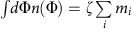is the total flux. Figure 3a,b shows that the mobility flux density follows a power-law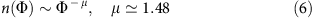holding for over seven orders of magnitude. Note that it actually holds for over nine orders of magnitude; however, we may neglect the very small flux values (below 10−4) as they do not contribute significantly to traffic. The scaling behaviour (6) can be derived from a counting argument using (1), described as follows. At intermediate to large ranges for cab, the population sab within the ameboid domain is much larger than those at sites a or b: sab >> max(ma, nb) and therefore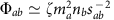. Assuming a typical population size ‹m› at any node, we have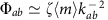, where kab is the number of nodes within the ameboid domain. Moreover, k= kab is also the index of the node on the minimal path tree centred on a (index 0) just before node b. As the index has a uniform distribution, we can use the method of inverse transform: Φ′(k)=−2ζmk−3, k0(Φ)=(ζm›)1/2Φ−1/2 so n(Φ) =1/|Φ′(k0)| =(1/2)(ζm›)1/2Φ−3/2, and thus μ=3/2. In Supplementary Note 2 we show that an alternative approach using the assumption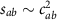and computing thus the distribution of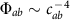, while also leading to a power law, generates an exponent of 1.3 (Supplementary Fig. 3). The reason for why this approach generates a different exponent for the flux distribution is because the assumptiondoes not hold for the roadway network due to the fractal-like nature21,22,38 of the ameboid domains; instead it obeys a scaling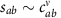with ν1.33 (Supplementary Fig. 4). This observation provides additional support to studies of the fractal morphology and the underlying roadway networks of urban sprawls21,39.

The scaling law (6) implies that over several orders of magnitude the OD fluxes are heterogeneous and scale-invariant, namely, fluxes from fractional values to hundreds of thousands of vehicles are transported across the highway network, daily. This, in turn determines the width of the traffic distribution, which, as shown in the following sections, obeys a log-normal distribution. The power-law (6) is a consequence of the scaling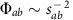, which in turn is a consequence of the threshold condition for mobility in the radiation law (Supplementary Note 1) that is, of the fact that individuals will travel to the site that meets their expectation and it is the least costly to reach on the network.

### Network flow modelling

The traffic values were computed on all edges using equations (2, 3, 4, 5) and compared with real traffic values on the subset of edges for which these data are available (red edges in Fig. 2a). Figure 4 shows the comparisons using the density of log traffic ρ(log10(T)) and the PCCs between data and model traffic values.

The case without capacity limitation is shown in Fig. 4a. The overall multiplying factor ζ in the model was set to match the mean of the distribution of traffic in the model with that in the data. As shown in the left panel of Fig. 4a, the model distributions (blue and red lines) track rather closely the log traffic distribution (black line) of the data with a slightly better agreement when using travel-time-based cost functions. The PCCs, however, show a significant difference, 0.273 versus 0.639, indicating that travel time is a much better criterion for evaluating cost of travel than travel distance. Although for the travel-distance-based model there are no other adjustable parameters, one could state that for the travel-time-based case, however, the velocities provide enough wiggle room to achieve the much better fit with the data. While indeed, the fit is improved by varying the velocities, this is not the main reason for the agreement. The typical travel velocities were obtained using a consistent procedure described in Supplementary Method 1. To avoid too many fitting parameters, we have not used separate velocities for individual roads, but all roads were lumped into three velocity ranks: fast, medium and within-city speeds. For the velocity combinations tested shown in Supplementary Table I, the corresponding PCCs were all found to be above 0.61, still much higher than the 0.27 PCC from the travel-distance-based model.

A better agreement can be achieved if capacity limitation is taken into consideration (Supplementary Method 2), see Fig. 4b. The distributions of the log traffic show an even better match, and the highest obtained PCC is 0.752 when using travel time costs. In the case of capacity limitation, the iterations were stopped when condition (5) was satisfied. Figure 5 shows roadway traffic values (using colours to indicate the volume of the traffic) for visual comparison between model and data, showing a relatively good agreement between the two, for most of the roads.

The traffic values were generated using the weighted betweenness centrality type expression (2). On the basis of this we can give an analytic argument for why the shape of the traffic density plotted in Fig. 6 is lognormal. It was previously shown25,37 that (for example equation (6) of ref. 37) the natural scaling variable for the betweenness distribution is the logarithm of the betweenness (hence traffic) and that the betweenness distribution can be written as a convolution between the degree distribution P(k) and the distribution function Ψr of the deviation (noise) of the shell sizes (the number of network nodes at a given range r) from its scaling form described by the corresponding branching process characteristic for that network class. That is, if b denotes the betweenness variable, p(b)~(1/b) ∫dk P(kr(log b−log βr−log k). For spatial networks such as random geometric graphs, or roadways, this scaling form is power-law with the exponent given by the dimensionality of the embedding space (d=2) that is βr~rd=r2. As our degree distribution is almost uniform we can make P(k)~δ(k−‹k›) with good approximation, which from above leads to p(b)~(1/br(log b−log βr−log‹k›). As shown in refs 25, 37 Ψr is Gaussian for large random networks (also for the US highway network), and thus the betweenness/traffic distribution becomes a lognormal, indeed supported by Fig. 6.

## Discussion

There are several gravity models in the literature that may be used to better match the local traffic, but they come at the expense of additional fitting parameters3,7,30,31,32. However, if we would need to predict new flow patterns in the wake of network changes (for example due to natural disasters) it is not clear what values should be used for the fitting parameters on the changed network. The main strength of our approach is that it is based on first principles and thus it can be easily used for flow predictions in the wake of network changes. The model can be further improved by adding more features such as a better approximation to population distribution at the intersection level, seasonal variations and so on. And indeed, we have seen the agreement improving already by including capacity limitations, even with crude approximations for travel speeds. At every step, our modelling approach follows the Maximum Entropy Principle by Jaynes40 in the sense that the model incorporates only known data (population distribution, the network and capacities) and the assumed behaviour (cost-based radiation law and cost minimizing path-choice); for everything else it assumes uniform distributions with minimum parameters so as to minimize biases (such as the coefficient ζ or the distributions within speed categories).

The original radiation model treats costs simply as a geometric range; it does not involve any transportation network. As our framework allows the use of any cost-function, we could still use the original radiation model for calculating the fluxes Φab by calculating the area populations sab using geodesic, or in this case, great-circle distances. However, we cannot use great-circle distances to find the lowest travel cost paths on the network because great-circle distances say nothing about network paths. Thus, we would be forced to employ two, somewhat inconsistent travel cost criteria: when estimating the area population that we can reach (sab) we would use as-crow-flies distances, but when computing network paths for travel we have to revert to network-based travel costs. This would lead to errors in geographically heterogeneous areas, where a direct path to a location may run through an obstacle (such as a lake, a mountain, a gorge and so on), and thus that location would be included into sab, but the real network path would avoid the obstacle at a more significant cost (excluding that location from sab). Statistically, however, using the original radiation model would not lead to large errors in the traffic distribution ρ and the PCC for a large country as the United States. The reason is because using great circle distances we still get a good approximation of the population sab on the network for most OD pairs (a, b). Both the PCC and the traffic distribution imply sums/averages taken over a large fraction of the whole United States, and these averages are dominated by short and medium distances, which are abundant in heavily populated areas. With some exceptions, heavily populated areas tend to be in regions where mobility is not hampered by geographical obstacles and thus in these heavily populated areas network paths tend to run in the direction of the shortest geometrical distance, making the two cost measures proportional to one another.

Besides consistency, our model also has a computational advantage in that we can simultaneously find the lowest cost paths and the population values sab (within the Dijkstra part of the algorithm, see the Methods section), within one run of the algorithm. However, when computing the fluxes Φab using great-circle distances we need a separate algorithm of an entirely different nature, which is in addition to the flux distribution code. This additional algorithm needs to find all the points increasingly by their great-circle distance from an origin a, then it needs to do this for all (N) origins. This is a well-known problem in computational geometry and the most efficient implementation runs in O(N2log N) time41. Thus, as the flux distribution algorithm is also of O(N2log N) complexity (the roadway network is sparse), this additional algorithm essentially doubles the computational time (confirmed by our simulations).

In summary, the cost-based radiation model provides a feasible approach to model flows in spatial networks where the choice of transport paths on the network is driven by a cost-minimization principle, given the distribution of population and resources. The mobility fluxes are generated by the individuals finding those absorption sites on the network that meet their expectation thresholds and that are the least costly to reach on the network. This couples the socio-demographic aspect (mobility law) with the network transport aspect (flux distribution), and the final flow will be the result of the interplay between these two aspects. Because of its principled nature, we expect that the modelling approach presented here is applicable with some modifications not just for highway network data sets but for spatial networks in general where traffic is generated by a cost-incurring transport.

## Methods

### Assigning populations to network vertices

To compute the mobility fluxes Φab we need to know not only the populations at sites a and b but also at all sites around a within the domain defined by the cost function cab. As we are modelling traffic at the level of road intersections, we need to resolve the distribution of population at this level. For this purpose, we used population information from the US government’s zipcode database (http://federalgovernmentzipcodes.us/). Restricted to the contiguous US, the corresponding population data came from 31,343 zip code instances. However, there are N=137,267 network vertices (intersections), which implies that a finer resolution is needed than what is provided by zip codes, for population. We perform this refinement in two steps. First, we construct a 2D Voronoi diagram using the set of points (Voronoi sites) provided to us in the zip code data (these usually correspond to post-office locations, given in (long, lat)) and assign every intersection (network node) to that Voronoi site to which it is the closest. Second, we label those Voronoi cells that had no intersections assigned to them (26%). We remove their sites temporarily, then we redo the Voronoi mesh with these labelled sites absent. Next we place back the labelled sites and find those Voronoi cells from the second mesh that contain these labelled sites. We then add the population of the labelled sites to the population of those cells from the second mesh that contain them, and redistribute the population among the intersections within all cells of the second mesh, uniformly, see Fig. 2b. This way no population is lost and they are all assigned naturally to the closest intersections.

### Weighted betweenness centrality algorithm

This algorithm proceeds by constructing the MPT rooted at a vertex a, for all vertices a using Dijkstra’s algorithm42 (based on breadth-first search). Then starting from the leafs (the furthest nodes from the root a) of the MPT it computes recursively for every edge (i, j) of the MPT the contributions in the sum (2) coming from all paths with source node a. Note that for a given root (source) node a only those fluxes Φ contribute to these sums for which ε is part of the corresponding MPT. Thus, we don’t need to generate all the fluxes Φab for all pairs beforehand (which would be on the order of 2 × 1010 values for the US highway system), but we can compute them locally when generating the minimum paths tree.

### Distributing flows in networks with capacity limitation

Consider the first step k=1. Denoting the whole-graph with G and its edge set by E, starting with G we compute the non-adjusted flow values tij;1tij(G) on all edges. We identify the set L1 of q roads with the smallest Cij/tij;1 ratio, which are the roads that become congested early on. Define: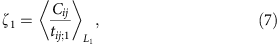where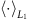is an average taken over the edges in L1. For edges in L1, ζ1tij;1 will be near their capacity Cij (if q is not too large). This allows for fluctuations around the congestion capacities, modelling the softness effect mentioned in the main text. The adjusted flow on edge (i, j) at the end of the first step will therefore be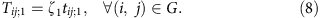On the non-congested edges (i, j)L1, the new capacity will be Cij;1=CijTij;1. In the next step (k=2) we consider the new graph G1 with edge-set E1=E\L1 (removed the q congested edges identified in the previous step). We then compute the non-adjusted flow tij;2=(1−ζ1)tij(G1) for all edges of G1. The latter corresponds to flow computed with mobility fluxes Φab=(1−ζ1)mapab because a ζ1 fraction of the population is already on the roads. We now identify the set L2E of q edges (|L2|=q) with the smallest ratios Cij;1/tij;2 and define: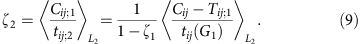Then, the new, adjusted flow on the edges of G1 will be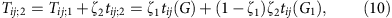(i, jG1, with the new capacities for further traffic becoming Cij;2=CijTij;2. In the third step k=3, we compute the non-adjusted flow tij;3=(1−ζ1)(1−ζ2)tij(G2), from fluxes Φab=(1−ζ1)(1−ζ2)mapab corresponding to the fraction of population not in the network, where G2 is obtained from G1 by removing the edges in L2. We then identify the set L3 of q edges with the smallest Cij;2/tij;3 ratios and compute: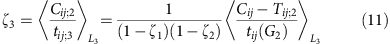yielding the adjusted flow on all the roads (i, j) of G2: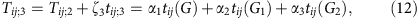(i, jG2, where α1=ζ1, α2=ζ2(1−ζ1), α3=ζ3(1−ζ2)(1−ζ1). Thus, in the first step we distributed ζ1m=α1m travellers, in the second step another (1−ζ1)ζ2m=α2m, in the third (1−ζ1)(1−ζ2)ζ3m=α3m and so on. A straightforward generalization of this yields the equations in the main text.

### Determining the effective range limitation

The very small mobility flux values in Fig. 3a,b are coming from OD pairs whose separation involves a large travel cost cab. However, we expect that fluxes that are too small (10−4 and smaller) do not contribute significantly to any traffic flow value, implying that we may limit our computaton of fluxes to ranges that generate fluxes that are not too small. To assess when range limitation is effective, we have computed the fraction of population from a location a travelling to sites whose travel cost (from a) is beyond a given threshold value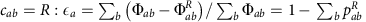, where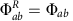if cabR and zero otherwise, and we used the expressions Φab=ζmapab and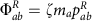. This fraction εa is the probability that a person from location a will travel beyond range R, which is then omitted from traffic flow calculations with range limit R. Supplementary Fig. 6a,b shows the cumulative fraction of the locations with long-range (larger than R) travel probability less than ε. When cost of travel is computed based on travel distance, we see that for 95% of all locations the likelihood of daily long-range travel is less than 1, 0.2 and 0.05% when going beyond 100, 200 and 400 km, respectively. In terms of travel time cost, 95% of all locations have less than 0.5, 0.09 and 0.02% likelihood of one-way daily trips taking longer that 100, 200 and 400 min, respectively. While neglecting these probabilities causes some error in the traffic values, the PCC (between flow data and model) saturates as function of the range limit, as shown in Supplementary Fig. 6c,d. In particular, at 100 km or 100 min the PCCs are already close to their corresponding saturation values. As in Fig. 3b, this translates back to about 10−4 below which mobility flux values can be neglected.

How to cite this article: Ren, Y. et al. Predicting commuter flows in spatial networks using a radiation model based on temporal ranges. Nat. Commun. 5:5347 doi: 10.1038/ncomms6347 (2014).

## References

1. Brockmann, D., Hufnagel, L. & Geisel, T. The scaling laws of human travel. Nature 439, 462–465 (2006).

2. Gonzalez, M. C., Hidalgo, C. A. & Barabási, A. L. Understanding individual human mobility patterns. Nature 453, 779–782 (2008).

3. Krings, G., Calabrese, F., Ratti, C. & Blondel, V. D. Urban gravity: a model for inter-city telecommunication flows. J. Stat. Mech. - Theory Exp. 7, L07003 (2009).

4. Onnela, J. P. et al. Structure and tie strengths in mobile communication networks. Proc. Natl Acad. Sci. USA 104, 7332–7336 (2007).

5. Eubank, S. et al. Modelling disease outbreaks in realistic urban social networks. Nature 429, 180–184 (2004).

6. Colizza, V., Barrat, A., Barthelemy, M. & Vespignani, A. The role of the airline transportation network in the prediction and predictability of global epidemics. Proc. Natl Acad. Sci. USA 103, 2015–2020 (2006).

7. Balcan, D. et al. Multiscale mobility networks and the spatial spreading of infectious diseases. Proc. Natl Acad. Sci. USA 106, 21484–21489 (2009).

8. Hill, D. J. & Chen, G. Power systems as dynamic networks.IEEE Int. Symp. Circuits and Systems 722–725 (2006).

9. Dörfler, F., Chertkov, M. & Bullo, F. Synchronization in complex oscillator networks and smart grids. Proc. Natl Acad. Sci. USA 110, 2005–2010 (2013).

10. Motter, A. E., Myers, S. A., Anghel, M. & Nishikawa, T. Spontaneous synchrony in power-grid networks. Nat. Phys. 9, 191–197 (2013).

11. Wilson, A. G. The use of entropy maximizing models in the theory of trip distribution, mode split and route split. J. Transp. Econ. Policy 3, 108–126 (1969).

12. Makse, H., Havlin, A. & Stanley, H. Modeling urban-growth patterns. Nature 377, 608–612 (1995).

13. Barrett, C. L. et al. TRANSIMS: Transportation Analysis Simulation System Technical Report LA-UR--00--1725Los Alamos National Laboratory (2001).

14. Wu, Z., Braunstein, L. A., Havlin, S. & Stanley, H. E. Transport in weighted networks: Partition into superhighways and roads. Phys. Rev. Lett. 96, 148702 (2006).

15. Bono, F., Gutiérrez, E. & Poljansek, K. Road traffic: a case study of flow and path-dependency in weighted directed networks. Physica A: Stat. Mech. Appl. 389, 5287–5297 (2010).

16. Barthelemy, M. Spatial networks. Phys. Rep. 499, 1–101 (2011).

17. Roth, C., Kang, S. M., Batty, M. & Barthelemy, M. Structure of urban movements: polycentric activity and entangled hierarchical flows. PLoS ONE 6, e15923 (2011).

18. Wang, P., Hunter, T., Bayen, A. M., Schechtner, K. & Gonzalez, M. C. Understanding road usage patterns in urban areas. Sci. Rep. 2, 1001 (2012).

19. Ercsey-Ravasz, M., Toroczkai, Z., Lakner, Z. & Baranyi, J. Complexity of the international agro-food trade network and its impact on food safety. PLoS ONE 7, e37810 (2012).

20. Krueckeberg, A. D. & Silvers, A. L. It Urban Planning Analysis: Methods and Models Wiley (1974).

21. Batty, M. The size, scale and shape of cities. Science 319, 769–771 (2008).

22. Batty, M. & Longley, P. A. Fractal Cities: a Geometry Of Form And Function Academic Press (1994).

23. Benenson, I. & Torrens, P. M. Geosimulation: Automata-based Modeling Of Urban Phenomena Wiley (2004).

24. Holme, P., Kim, B. J., Yoon, C. N. & Han, S. K. Attack vulnerability of complex networks. Phys. Rev. E 65, 056109 (2002).

25. Ercsey-Ravasz, M., Lichtenwalter, R. N., Chawla, N. V. & Toroczkai, Z. Range-limited centrality measures in complex networks. Phys. Rev. E 85, 066103 (2012).

26. Carter, M. R. et al. Effects of Catastrophic Events on Transportation System Management and Operations, The Pentagon and the National Capital Region. U.S. Department of Transportation Technical Report Cambridge, MA, USA (2003).

27. Simini, F., Gonzalez, M. C., Maritan, A. & Barabási, A. L. A universal model for mobility and migration patterns. Nature 484, 96–100 (2012).

28. Zipf, G. K. The p1 p2/d hypothesis: on the intercity movement of persons. Am. Sociol. Rev. 11, 677–686 (1946).

29. Erlander, S. & Stewart, N. F. The Gravity Model in Transportation Analysis: Theory and Extensions VSP (1990).

30. Jung, W. S., Wang, F. & Stanley, H. E. Gravity model in the korean highway. Europhys. Lett. 81, 48005 (2008).

31. Kaluza, P., Koelzsch, A., Gastner, M. T. & Blasius, B. The complex network of global cargo ship movements. J. R. Soc. Interface 7, 1093–1103 (2010).

32. Viboud, C. et al. Synchrony, waves, and spatial hierarchies in the spread of influenza. Science 312, 447–451 (2006).

33. Simini, F., Maritan, A. & Néda, Z. Human mobility in a continuum approach. PLoS ONE 8, e60069 (2013).

34. Brandes, U. A faster algorithm for betweenness centrality. J. Math. Sociol. 25, 163–177 (2001).

35. Newman, M. Scientific collaboration networks. II. Shortest paths, weighted networks, and centrality. Phys. Rev. E 64, 016132 (2001).

36. Sreenivasan, S., Cohen, R., López, E., Toroczkai, Z. & Stanley, H. E. Structural bottlenecks for communication in networks. Phys. Rev. E 75, 036105 (2007).

37. Ercsey-Ravasz, M. & Toroczkai, Z. Centrality scaling in large networks. Phys. Rev. Lett. 105, 038701 (2010).

38. Makse, H. A., Havlin, S. & Stanley, H. E. Modeling urban-growth patterns. Nature 377, 608–612 (1995).

39. Bettencourt, L. M. A., Lobo, J., Helbing, D., Kühnert, C. & West, G. B. Growth, innovation, scaling and the pace of life in cities. Proc. Natl Acad. Sci. USA 104, 7301–7306 (2007).

40. Janes, E. T. Information theory and statistical mechanics. Phys. Rev. Ser II 106, 620–630 (1957).

41. Dickerson, M. T. & Eppstein, D. Algorithms for proximity problems in higher dimensions. Comp. Geom. Theo. App. 5, 277–291 (1996).

42. Dijkstra, E. W. A note on two problems in connexion with graphs. Numerische Mathematik 1, 269–271 (1959).

## Acknowledgements

We thank A.L. Barabási, Z. Néda and H.T. Wang for discussions. We also thank R. Lychtenwalter for his computational help to speed up the betweenness algorithm, and Sz. Horvát and M. Varga for a critical reading of the manuscript. This work was supported in part by US HDTRA 1-09-1-0039 (M.E.-R., Y.R. and Z.T.), the US NSF BCS-0826858, in part by grant FA9550-12-1-0405 from the U.S. Air Force Office of Scientific Research and Defense Advanced Research Projects Agency (Z.T.) and by a grant of the Romanian CNCS-UEFISCDI, Project No. PN-II-RU-TE-2011-3-0121 (M.E.-R.).

## Author information

Authors

### Contributions

Y.R. analysed data, wrote simulation software, contributed tools; M.E.-R. contributed tools and designed methods; P.W. and M.C.G. provided, prepared and analysed data. Z.T. designed the research and wrote the paper.

### Corresponding author

Correspondence to Zoltán Toroczkai.

## Ethics declarations

### Competing interests

The authors declare no competing financial interests.

## Supplementary information

### Supplementary Information

Supplementary Figures 1-6, Supplementary Tables 1-2, Supplementary Notes 1-2, Supplementary Methods 1-2 and Supplementary References (PDF 5504 kb)

## Rights and permissions

Reprints and Permissions

• ### Kernel-based formulation of intervening opportunities for spatial interaction modelling

• Masaki Kotsubo
• Tomoki Nakaya

Scientific Reports (2021)

• ### The education-chasing labor rush in China identified by a heterogeneous migration-network game

• Xiaoqi Zhang
• Yanqiao Zheng
• Zhan Chen

Scientific Reports (2020)

• ### A universal opportunity model for human mobility

• Er-Jian Liu
• Xiao-Yong Yan

Scientific Reports (2020)

• ### Estimation of Regional Economic Development Indicator from Transportation Network Analytics

• Bin Li
• Song Gao
• Runmou Xiao

Scientific Reports (2020)

• ### Universal scaling laws of collective human flow patterns in urban regions

• Yohei Shida
• Hideki Takayasu
• Misako Takayasu

Scientific Reports (2020)